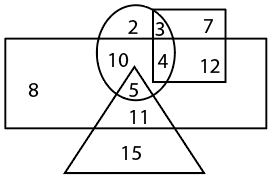Question 19

# In the Venn diagram given below. the circle represents 'North Indian'. the triangle represents 'Pianists', the rectangle represents 'Males' and the square represents 'Engineers'. The numbers given in the diagram represents number of persons of that particular category. Who among the following is a female, North Indian; Engineer?Solution

Given that the circle represents 'North Indian'. the triangle represents 'Pianists', the rectangle represents 'Males' and the square represents 'Engineers'

the Venn diagram is given belowthen from the given Venn diagram a female, North Indian; Engineer = 3 ( circle represent North Indian and square represent to Engineers )

therefore option (C) 3 Ans

• Free SSC Study Material - 18000 Questions
• 230+ SSC previous papers with solutions PDF